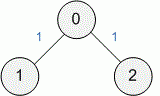Competitions

# Tree

Time limit 1 second
Memory limit 128 MiB

A weighted tree is given. Find the distance between two given vertices.

## Input data

The first line contains the number of nodes n~(1 \le n \le 150000) in the tree. The nodes are numbered from 0 to n - 1. Each of the next n - 1 lines contains three integers u, v, w that correspond to an edge with weight w~(0 \le w \le 1000), connecting nodes u and v. The next line contains the number of queries m~(1 \le m \le 75000). In each of the next m lines there are two integers.

## Output data

For each query print the distance between the nodes with the given numbers.## Examples

Input example #1
3
1 0 1
2 0 1
3
0 1
0 2
1 2

Output example #1
1
1
2

Author Dmitry Zhukov
Source 2006 Petrozavodsk Summer Session, Ural SU and Orel STU Contest, August